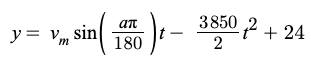Search
• Sajiv Shah

# FRC 2021 Infinite Recharge @ Home Strategy Part 4: Estimating Shot Trajectories

Since making shots into the three-point zone is crucial to winning games, it also drives our robot design. We already determined two major locations where we will be able to take uncontested shots, meaning that we should design our shooter to ensure we can make shots from those locations. To do this, we used simple graphs and kinematics.

The setup of the goals was simple. We needed to determine the distances and heights in inches using the field CAD, and then simply represented them as lines in the graph. Though 2d, this graph accounts for the entire length of the shot, and also ensures that shots can make it through the sides of the hexagonal outer port.Let's look at how the parametric equation of the shot trajectory was modeled. This graph assumes a basic 2d kinematics situation in which there is only one net force acting on the object, which is gravity. Obviously, in reality, air resistance in both the vertical and horizontal direction would impact this trajectory, but because those factors cannot be calculated for without extensive physics knowledge or prototyping, we excluded them. That being said, height can simply be modeled by:where (v) is the vertical component of the velocity and (a) is the acceleration due to gravity, and (t) is time. In our case, v is simply represented by the velocity of the ball multiplied by the sin of the angle, and (a) is gravity in inches/seconds^2, which is constant at 3850. Implementing this equation into our desmos calculator gives us:the variable a is an angle that is in the range from 0-90, which is then converted to radians in the equation. The y-intercept of 24 is to account for the initial height that the ball is shot from. To represent the trajectory of the shot, simply graphing our x-axis as the time (t) will not work as that will instead give us a position vs. time graph. Instead, we must find the relationship between (x) and (t) and use a parametric equation to graph (x) vs. (y). The equation below comes from a basic d=rt equation as there is no net force in the horizontal direction.Using this, we can then generate a trajectory with two sliders. One for the exit velocity of the ball from the shooter (v) and the other for the angle of the shot (a). These two variables drive whether our shooter will need to have a variable wheel speed, which from a software perspective makes the system complex, or if it needs a variable hood angle, which makes the design complicated from a hardware and software perspective, and finally if we need to add the ability to change the height of the shooter. In the next post, we will use these trajectories to analyze what variables we need to change to make shots into the three-point goal from multiple locations.

See All